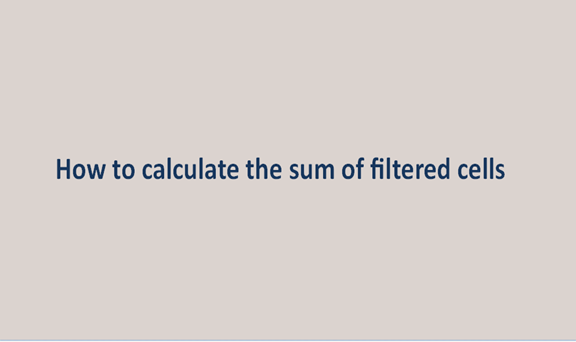## How to calculate the sum of filtered cells

When one wants to hide data from the view, excel has a filter option that filters data in the worksheet. This also data into the filtered and the visible. It may be easy to sum numbers in an excel column, and sometimes you may need the filter option to hide some data to meet your objectives.

When you want to perform calculations in these cells, e.g., the sum of function values, including hidden data, will be added. The sum function does not know what values you want to evaluate and which not to now what do you do to add up only the filtered or visible data?

Method 1: Use of subtotal function

This subtotal function method ignores rows that a filter has excluded, and now only visible cells can be added up. This is done as follows:

Come up with the list of the table as I have done:1. In a blank cell, C13, for example, enter this formula: =Subtotal(109,C2:C5) (109 indicates when you sum the numbers, the hidden values will be ignored; C2:C5 is the Range you will sum ignoring filtered rows.), and press the Enter key.2. The sum of visible cells can be calculated using the formula above if the worksheet contains hidden rows. Ignoring cells in hidden columns cannot be worked out with the stated above formula.

Method 2: Use of user-defined functions

This works best with those who have gone much into the coding thing. Involves the use of visual basic.

1. Press the shortcut key alt+f11, which in turn will open the visual basic.

2. In the visual basic, go to "insert" then "Module" and have the following codes

Function SumVisible(WorkRng As Range) As Double

'Update 20130907

Dim rng As Range

Dim total As Double

For Each rng In WorkRng

Ifrng.Rows.Hidden=FalseAndrng.Columns.Hidden=FalseThen

total=total+rng.Value

End If

Next

SumVisible = total

End Function3. Save the code and enter formulae =SumVisible(C2:C5) on the empty cellMethod 3: Using kutoolcalculate, the sum of filtered cells

Usually, cells that are either filtered/hidden or not are counted in the specified Range using the SUM/Count/Average function. While ignoring hidden rows can only enable either summing or counting, or averaging. However, Kutools for Excel

SUMVISIBLE COUNTVISIBLE AVERAGEVISIBLE functions easily calculate the specified Range by ignoring any hidden cells, rows, or columns.

For those who cannot remember the formulae, download the Kutools from the web below,